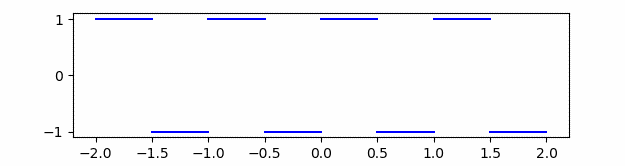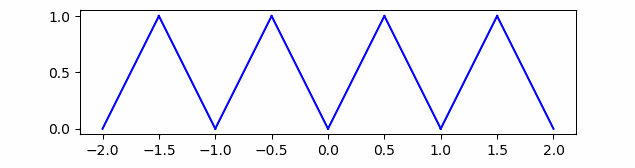# Square wave, triangle wave, and rate of convergenceThere are only a few examples of Fourier series that are relatively easy to compute by hand, and so these examples are used repeatedly in introductions to Fourier series. Any introduction is likely to include a square wave or a triangle wave.

By square wave we mean the function that is 1 on [0, 1/2] and -1 on [1/2, 1], extended to be periodic.Its nth Fourier (sine) coefficient is 4/nπ for odd n and 0 otherwise.

By triangle wave we mean 1 – |2x – 1|, also extended to be periodic.Its nth Fourier (cosine) coefficient is -2/(nπ)² for odd n and 0 otherwise.

This implies the Fourier coefficients for the square wave are O(1/n) and the Fourier coefficients for the triangle wave are O(1/n²). (If you’re unfamiliar with big-O notation, see these notes.)

In general, the smoother a function is, the faster its Fourier coefficients approach zero. For example, we have the following two theorems.

1. If a function f has k continuous derivatives, then the Fourier coefficients are O(1/nk).
2. If the Fourier coefficients of f are O(1/nk+1+ε) for some ε > 0 then f has k continuous derivatives.

There is a gap between the two theorems so they’re not converses.

If a function is continuous but not differentiable, the Fourier coefficients cannot decay any faster than 1/n²), so the Fourier coefficients for the triangle wave decay as fast as they can for a non-differentiable function.